Courses

# Metal Oxide Semiconductor Field Effect Transistor (MOSFET) Capacitor (Part - 1) Electrical Engineering (EE) Notes | EduRev

## VLSI System Design

Created by: Machine Experts

## Electrical Engineering (EE) : Metal Oxide Semiconductor Field Effect Transistor (MOSFET) Capacitor (Part - 1) Electrical Engineering (EE) Notes | EduRev

The document Metal Oxide Semiconductor Field Effect Transistor (MOSFET) Capacitor (Part - 1) Electrical Engineering (EE) Notes | EduRev is a part of the Electrical Engineering (EE) Course VLSI System Design.
All you need of Electrical Engineering (EE) at this link: Electrical Engineering (EE)

Objectives

In this course you will learn the following

• MOS as Capacitor
• Modes of operation
• Capacitance calculation of MOS capacitor

4.1 MOS as Capacitor

Refering to fig. 4.1, we can see there is an oxide layer below the Gate terminal. Since oxide is a very good insulator, it contributes to an oxide capacitance in the circuit.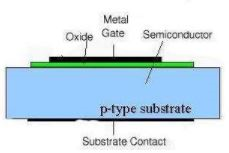Fig 4.1: Cross-section view of MOS Capacitor

Normally, the capacitance value of a capacitor doesn't change with values of voltage applied across its terminals. However, this is not the case with MOS capacitor. We find that the capacitance of MOS capacitor changes its value with the variation in Gate voltage. This is because application of gate voltage results in band bending in silicon substrate and hence variation in charge concentration at Si-SiO2 interface.

4.2 Modes of operation

Depending upon the value of gate voltage applied, the MOS capacitor works in three modes :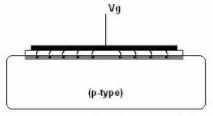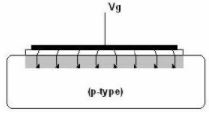Fig 4.2a: Accumulation mode (grey layer - strong hole concentration)   Fig 4.2b: Depletion Mode (light grey layer - depletion region)

1. Accumulation: In this mode, there is accumulation of holes (assuming n-MOSFET) at the Si-SiO2 interface. All the field lines emanating from the gate terminate on this layer giving an effective dielectric thickness as the oxide thickness (shown in Fig. 4.2a). In this mode, Vg <0

2. Depletion: As we move from negative to positive gate voltages the holes at the interface are repelled and pushed back into the bulk leaving a depleted layer. This layer counters the positive charge on the gate and keeps increasing till the gate voltage is below threshold voltage. As shown in Fig. 4.2b we see a larger effective dielectric length and hence a lower capacitance.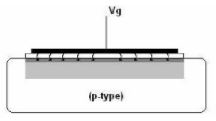Fig 4.2c: Strong Inversion mode (grey layer - strong electron concentration, light grey - depletion region)

3. Strong Inversion: When Vg crosses threshold voltage, the increase in depletion region width stops and charge on layer is countered by mobile electrons at Si-SiO2 interface. This is called inversion because the mobile charges are opposite to the type of charges found in substrate. In this case the inversion layer is formed by the electrons. Field lines hence terminate on this layer thereby reducing the effective dielectric thickness as shown in Fig. 4.2c)

4.3 Capacitace calculation of MOS Capacitor

In the last chapter, we gave you an introduction of MOS as capacitor. In this chapter, we will see how MOS works as a capacitor with derivation of some related equations.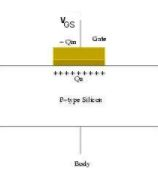Fig 4.3: Gate and Depeletion charge of MOS Capacitor

By Gauss's Law: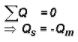Also by thermal equilibrium: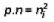where p and are hole and electron concentrations of substrate and ni is hole or electron concentration of the corresponding intrinsic seminconductor.

We see that if we keep making VGS more and more -ve, the charges Qs and Qm keep increasing. Thus, it is acting like a good parallel plate capacitor. Its capacitance can be given as-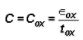For +ve bias voltage on gate, increasing VGS will increase Qm and Qs.

Using the depletion approximation, we can write depletion width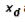as a function of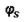as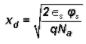where Na is the substrate acceptor density,∈s is dielectric constant of substrate andis the surface potential at substrate.

The depletion region grows with increased voltage across the capacitor until strong inversion is reached. After that, further increase in the voltage results in inversion rather than more depletion. Thus the maximum depletion width is: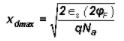Also,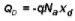Therfore at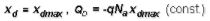But by Gauss's law, electrons must compensate for increasing Qs.

So,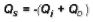where charge Qi is due to electrons in the inversion layer.

Earlier due to low electric field, the electron-hole pairs formed below the oxide interface recombine. However, once the electric field increases, the electron-hole pairs formed are not able to recombine. So the free electron concentration increases.

By Kirchoff's law, VGS  is given by: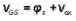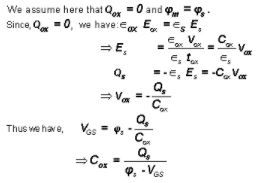Offer running on EduRev: Apply code STAYHOME200 to get INR 200 off on our premium plan EduRev Infinity!

35 docs|28 tests

,

,

,

,

,

,

,

,

,

,

,

,

,

,

,

,

,

,

,

,

,

;